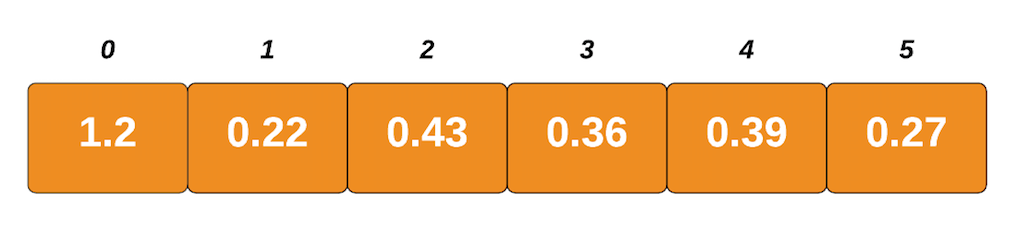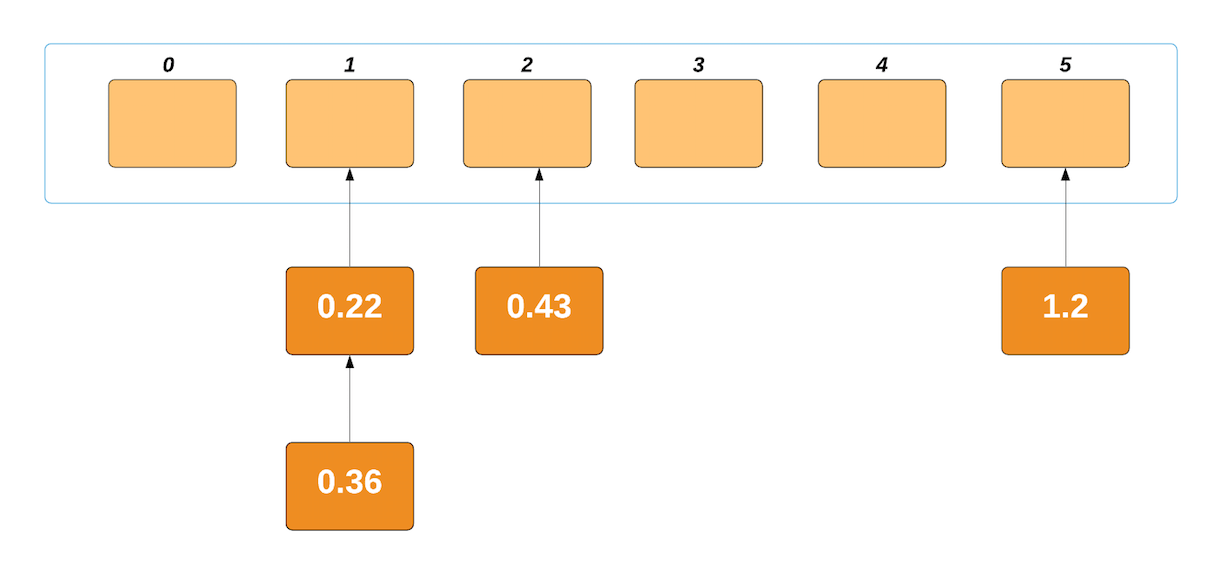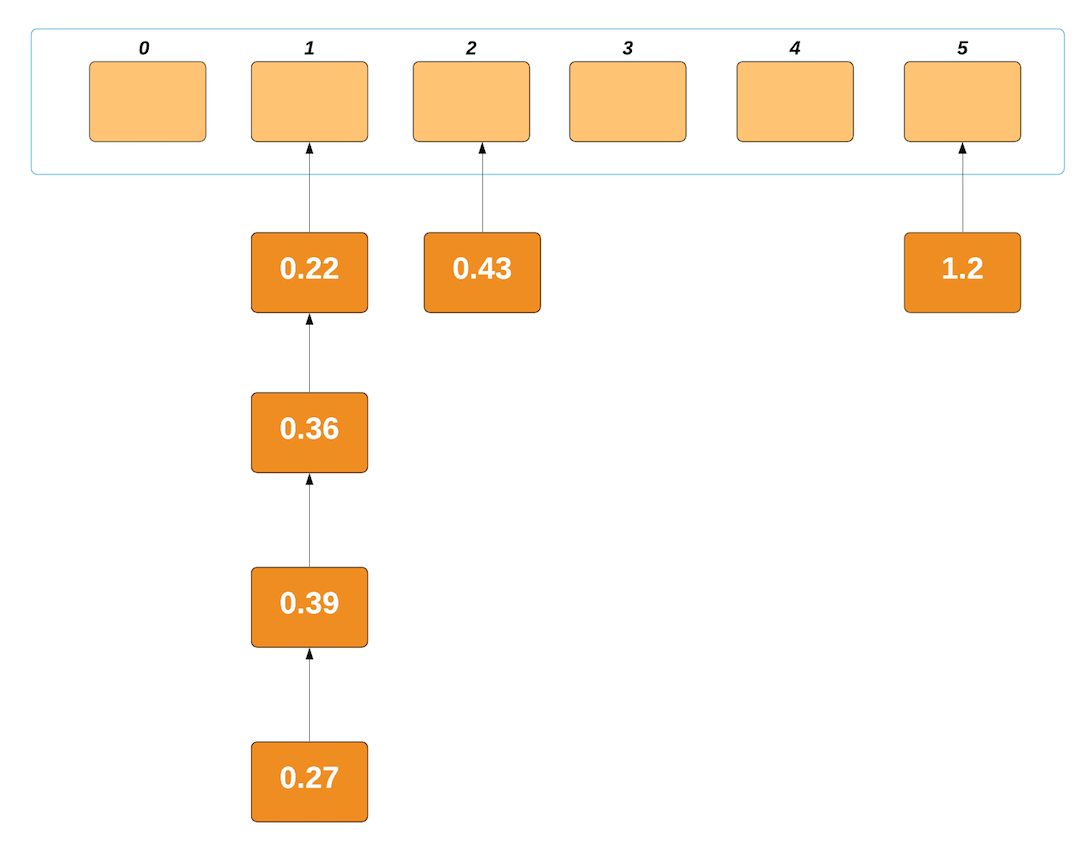# Bucket Sort Algorithm & Time Complexity | Python Implementation

## Introduction

In this tutorial, we are going to learn about a sorting algorithm named Bucket Sort. Bucket sort, or bin sort, is a comparison sort algorithm that works by assigning the elements of an array or list to a number of buckets. The elements within each bucket are then compared to each other and sorted individually by either recursively calling the Bucket sort function or by using a different sorting algorithm. In our example, we will be using the Insertion Sort as our intermediate algorithm to sort the elements within the individual buckets.

Bucket sort can be thought of as a scatter-order-gather approach towards sorting a list. Firstly, the elements of the input array or list are scattered into different buckets. Then, the elements are ordered or sorted within the containing bucket. Finally, the elements are gathered in order. This sorting approach is most suitable when the input is uniformly distributed over a range and also when you have data in decimals or floating-point values. For example, if you come across a problem that asks you to sort a large set of floating-point numbers, in the range from e.g. 0.5 to 1.5, uniformly distributed across the range, then Bucket sort is the best algorithm to do so.

### What to expect?

So basically, in this tutorial, we will be implementing Bucket sort on a list using Python. We will give you a step by step demonstration of how Bucket sort actually works. We will also discuss the computational and time complexities of the worst, average and best cases of the algorithm and compare Bucket sort’s performance with other sorting algorithms. Also, we will talk about some of the applications of Bucket sort itself. So, let’s begin!

## Prerequisites

To follow this tutorial, you should have some prior basic programming knowledge in any language (preferably in Python). Other than that, the rest of the article is pretty beginner friendly. We will be using Sublime Text for writing our implementation of the Bucket sort in Python 3. Feel free to use any other code editor that you like.

## Bucket Sort Algorithm

Before jumping into its exact implementation, lets walk through the algorithm a little bit. Bucket sort works as follows:

1. Set up a list of initially empty buckets. Number of buckets is equal to the length of the input list.
2. Go over the original list, putting each element in its bucket. This is determined by the length of the input list and the largest element in the input list. This will be clearly demonstrated in the example that follows.
3. Sort each non-empty bucket. We have used Insertion sort for this. You can use any other sorting algorithm for this as well.
4. Visit the buckets in order. This will yield a list as the output in which the elements are arranged from smallest to largest.

### Step by step demonstration of Bucket Sort Algorithm

1. Suppose the input list is:Largest number = 1.2
Length of list = 6
Size = Largest number / Length of list = 1.2/6 = 0.19999 = 0.2
This information will be useful in determining in which bucket will each element of the list be placed.

1. Create empty buckets. The number of buckets is equal to the length of list which in our case is 6. So, we will have 6 empty buckets.
1. Insert elements into the buckets from the list. The question is, how do we know in which bucket to place each element? Remember we calculated ‘size’ in the first step. We will basically divide each value of the list by size which is 0.2. The output will tell us the index of the bucket where the value is to be placed. Let’s consider our input list.

1.2 / 0.2 = 6. We will subtract 1 from this as it is outside of your index range. So, 6 – 1 = 5
Thus, the value 1.2 will be placed inside the bucket at index 5.

Likewise,

0.22/ 0.2 = 1.1 = 1. Thus, the value 0.22 will be placed inside the bucket at index 1.0.43/0.2 = 2.15 = 2. Thus, the value 0.43 will be placed inside the bucket at index 2.0.36/0.2 = 1.8 = 1 (Remember that we always consider the floor of our value. Thus 1.8 will not be rounded to 2, rather it will be rounded to 1). The value 0.36 will be placed inside the bucket at index 1.0.39/0.2 = 1.95 = 1. Thus, the value 0.39 will be placed inside the bucket at index 1.Lastly,
0.27/0.2 = 1.35 = 1. Thus, the value 0.27 will be placed inside the bucket at index 1. So, what we finally get after this step looks something like this:1. The elements within each bucket are now sorted using any of the stable sorting algorithms. Here, we have opted for Insertion sort. We will not be going into the details of this algorithm as our main focus is on Bucket sort algorithm. We will just talk briefly on how Insertion sort works and why we have chosen it as our intermediate sorting algorithm in one of the following sections. All you need to know for now is that after insertion sort, the elements within the individual buckets are sorted. They look something like this:
1. Lastly, the elements from each bucket are gathered or concatenated. It is done by iterating through each bucket and inserting individual elements into a list. This results in a final list with sorted elements which look like this:

### Python Code

``````    def bucketSort (input_list):
# Find maximum value and length of the input list to determine which value in the list goes into which bucket
largest = max (input_list)
length = len (input_list)
size = largest/length

# Create n empty buckets where n is equal to the length of the input list
buckets = [[] for _ in range (length)]

# Put list elements into different buckets based on the index
for i in range (length):
j = int (input_list[i] / size)

if j != length:
buckets[j].append (input_list[i])
else:
buckets[length - 1].append (input_list[i])

# Sort elements within the buckets using Insertion sort
for i in range (length):
insertionSort (buckets[i])

# Concatenate buckets with sorted elements into a single list
final_output = []
for i in range (length):
final_output = final_output + buckets[i]

return final_output

# Performs insertion sort algorithm on the individual bucket lists
def insertionSort (bucket_list):
for i in range (1, len (bucket_list)):
var = bucket_list[i]
j = i - 1
while (j >= 0 and var < bucket_list[j]):
bucket_list[j + 1] = bucket_list[j]
j = j - 1
bucket_list[j + 1] = var

def main ():
input_list = [1.20, 0.22, 0.43, 0.36,0.39,0.27]
print ('Original list: ', end = '')
print (input_list)
sorted_list = bucketSort (input_list)
print ('Sorted list: ', end = '')
print (sorted_list)

main ()``````

Output:

``````Original list: [1.2, 0.22, 0.43, 0.36, 0.39, 0.27]
Sorted list: [0.22, 0.27, 0.36, 0.39, 0.43, 1.2]``````

### Intermediate Sorting Algorithm within Bucket Sort

As discussed above, for sorting the elements in each of the individual buckets, we used the insertion sort algorithm. Why? because insertion sort’s runtime is based on how far each element is from its final position. This implies that the number of comparisons between elements remains relatively small, and the memory hierarchy is better exploited or utilized.

Insertion Sort’s fine details are outside the scope of this tutorial. Its functioning can be understood by looking closely at the insertionSort function in the above code. It takes the first element of bucket list and assumes it’s sorted. Then the second element is stored in the ‘var’ variable. It compares var with the first element; if the first element is greater than var, var is placed in front of first element such that the first two elements become sorted. It then takes the third element and compares it with elements on the left of it. The third element is placed just behind the element smaller than it. If there is no smaller element, it is placed at the beginning of the list. Similarly, every unsorted element is placed at its correct position. The overall output is the different bucket lists, with elements arranged from smallest to largest in each.

### Complexity

The complexity of an algorithm is simply the amount of work the algorithm performs to complete its task

#### Worst Case Complexity

Worst case occurs when the elements in the input list itself are arranged in the reverse order. When there are elements of close range in the list, which is the typical case for Bucket sort, they are likely to be placed in the same bucket and this may result in some buckets having a greater number of elements than others. This makes the complexity depend on the sorting algorithm used to sort the elements of the bucket, which in our case is insertion sort. Thus, if insertion sort is used to sort elements of the bucket, the time complexity for the worst case becomes O(n2).

#### Average Case Complexity

It occurs when the elements are distributed randomly in the list. Bucket sort runs in linear time in all cases until the sum of the squares of the bucket sizes is linear in the total number of elements. This makes O(n) to be the average case complexity of Bucket sort algorithm.

#### Best Case Complexity

Best case occurs when the elements in the input list itself are arranged in the correct order. Also, in the case of Bucket sort, if the elements are uniformly distributed within buckets, i.e. each bucket has the same number of elements as the other. If insertion sort is used to sort elements of a bucket, then the overall complexity in the best case will be linear i.e. O (n+k). O (n) is the complexity for making the buckets and O(k) is the complexity for sorting the elements of the bucket using algorithm having linear time complexity at best case.

### Comparison with other sorting algorithms

#### Counting sort:

Bucket sort can be converted to Counting sort if we reduce the size of each bucket to 1, i.e. each bucket can only hold one value. The variable bucket size of Bucket sort allows it to use O (n) memory instead of O(M) memory, where M is the number of distinct values as is the case in Counting sort. Therefore, Bucket sort gives up Counting sort’s O (n + M) worst-case behavior.

#### Merge sort:

In Merge sort, a list is distributed into several sub lists to be sorted. However, because of the overlapping value ranges, the sub lists cannot be combined to one as in the final step of the Bucket sort algorithm. Instead, they must be interleaved by a merge algorithm which adds complexity to the algorithm as compared to Bucket sort.

#### Quick sort:

Bucket sort with two buckets is a form of Quicksort where the pivot value is always selected to be the middle value of the value range. This choice is effective for uniformly distributed inputs, but for other means of choosing the pivot in Quicksort e.g. randomly selected pivots, this makes it more resistant to clustering in the input distribution.

### Bucket Sort Applications

• Computing histograms in data visualization and analysis. For example, n students might be assigned test scores in the range from 0 to 10 and are then placed into ranges or buckets on the basis of those scores.
• Working on data that is uniformly distributed over a close range
• Working on floating point or decimal values

### Conclusion

To sum it all up, we started off by getting an introduction to what Bucket sort is and went on to discuss what we need to know before we jump into its implementation in Python. We discussed its algorithm and each and every step involved in the sorting process. We talked about Insertion sort and why we chose it as our intermediate algorithm instead of other algorithms. Then the worst, average and best-case complexities of Bucket sort were analyzed. We drew a comparison and a relationship of Bucket sort with other sorting algorithms like counting, merge, and quick sort. Lastly, we covered some common applications of Bucket Sort. Overall, Bucket Sort is an important concept to understand when it comes to algorithms especially if you need to sort a list that is huge to fit into memory.